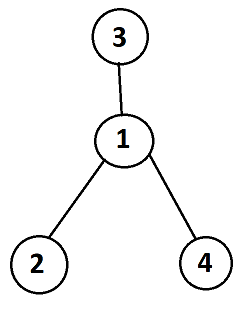# Distinct ListshefinAda Lovelace National Gir...
Limits 5s, 256 MB

Byteland has a network of $n$ computers that forms a tree structure. A tree is a connected graph that has no cycles.

Each computer $u$ has a special database and special software. The database has initially a list $L_u$. The list $L_u$ has only one element $A_u$ initially. The software is programmed to do a special task. When a list $B$ is given to the computer $u$’s special software, it starts to iterate through the list $B$. For each element $B_i$ of the list $B$, the software does the following:

• If the element $B_i$ already exists in the list $L_u$ the software does nothing. Otherwise, it appends $B_i$ to the end of the list $L_u$.

After completing the iteration, the software then sorts the list $L_u$ in ascending order. For example, if a list $L_u$ is $$ initially and we give the lists $[13, 75, 26], [0, 7], [0, 7, 13, 25]$ to the software of computer $u$ one by one, then eventually the list $L_u$ will be $[0, 7, 13, 25, 26, 75]$.

To ensure the security of the network, each computer sends a copy of all the lists in its database to its parent computer (if exists) only. When a computer $u$ gets the lists from a child computer, the computer $u$ adds the lists to its database and also gives the lists to its special software to update the list $L_u$. Only after receiving all the lists from all the children's computers and updating the list $L_u$ accordingly, a computer $u$ sends a copy of all the lists in its database to its parent computer (if exists).

For each computer $u$, you have to tell how many distinct lists are present in its database after completing all the above operations. Two lists are different if either they have different sizes or one list has at least one element that is not present in the other list.

## Input

Input starts with an integer $T(1\leq T\leq 10^5)$, the number of test cases.

In each test case, the first line contains an integer $n(1\leq n\leq 10^5)$, the number of computers.
The next line contains $n$ space-separated integers $A_i(0\leq A_i\leq 10^9)$, the single initial element of the list $L_i$ of computer $i$.
The last line of each test case contains $n$ space-separated integers $P_i(1\leq P_i\leq n)$, the parent computer of computer $i$. If computer $i$ is the root of the tree, $P_i$ will be $-1$.

It is guaranteed that the computer network will form a tree structure and the sum of $n$ over all test cases will not be greater than $10^6$. It is also guaranteed that if $n>10^4$, then $T=1$.

## Output

In each test case, print $n$ space-separated integers in a line. The $i^{th}$ integer represents the number of distinct lists present in the database of the computer $i$.

## Sample

InputOutput
2
4
7 10 15 10
3 1 -1 1
3
25 25 25
-1 1 1

2 1 3 1
1 1 1


Notes:

In the first test case, the computer network looks like the below tree where the computer $3$ is the root of the tree:The only list in computer $2$’s database is $$ which is also the list $L_2$. It is the only distinct list on this computer.
The only list in computer $4$’s database is $$ which is also the list $L_4$. It is the only distinct list on this computer.
The lists in computer $1$’s database are: $[7,10], , $ where the first list is the list $L_1$. The two distinct lists are: $[7,10], $.

The lists in computer $3$’s database are: $[7,15,10], [7,10], , $ where the first list is the list $L_3$. The three distinct lists are: $[7,15,10], [7,10], $.

### Submit